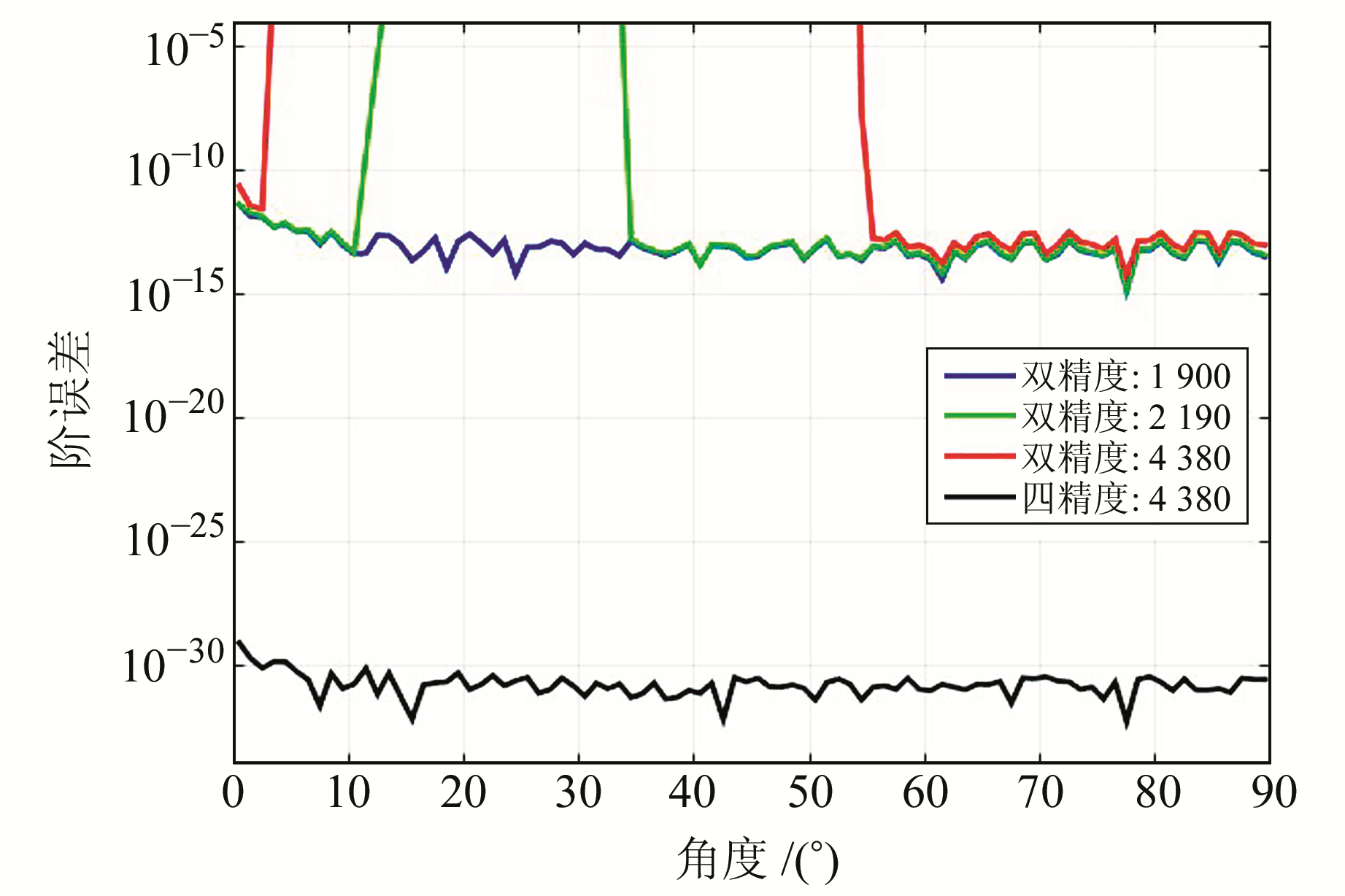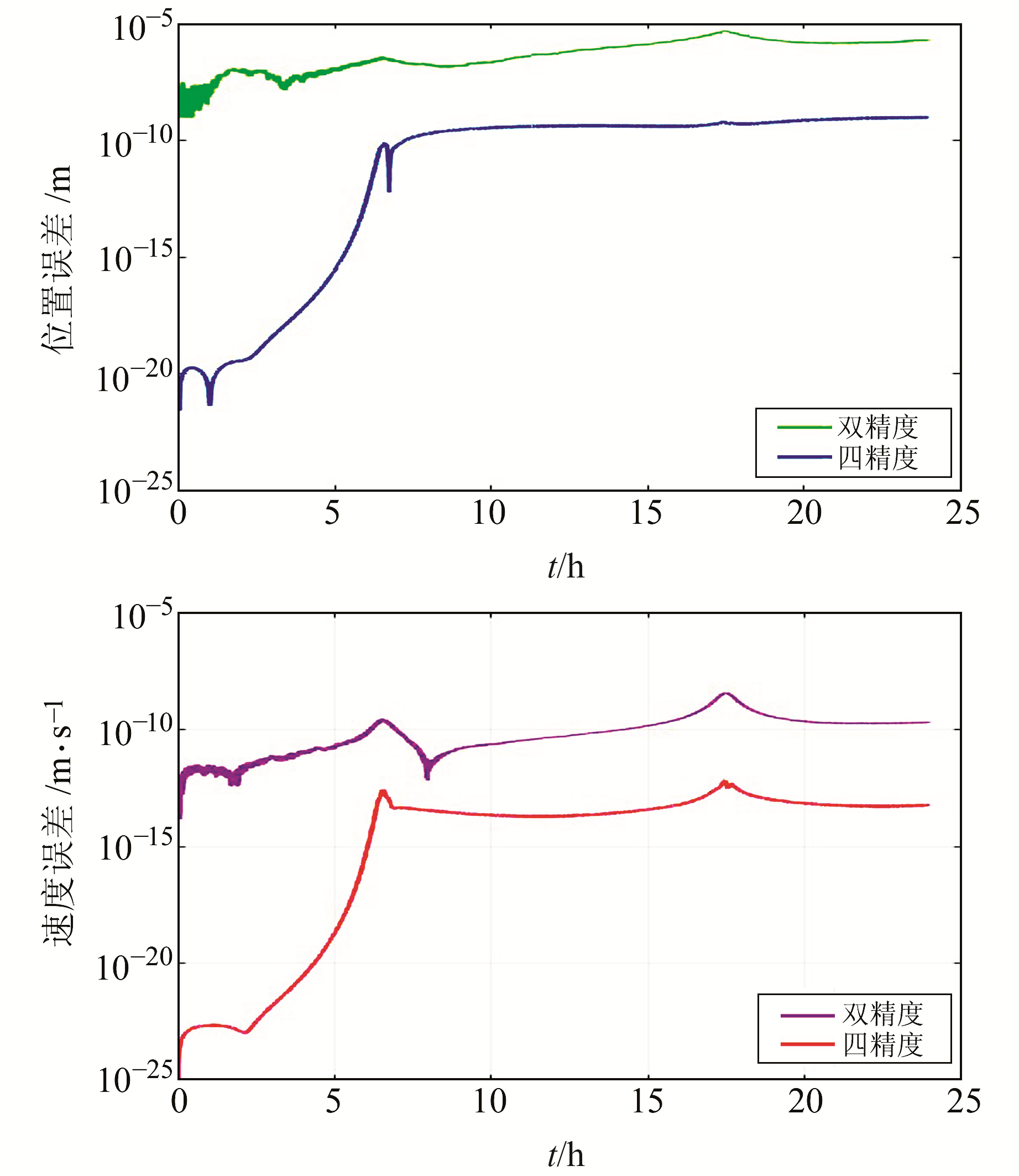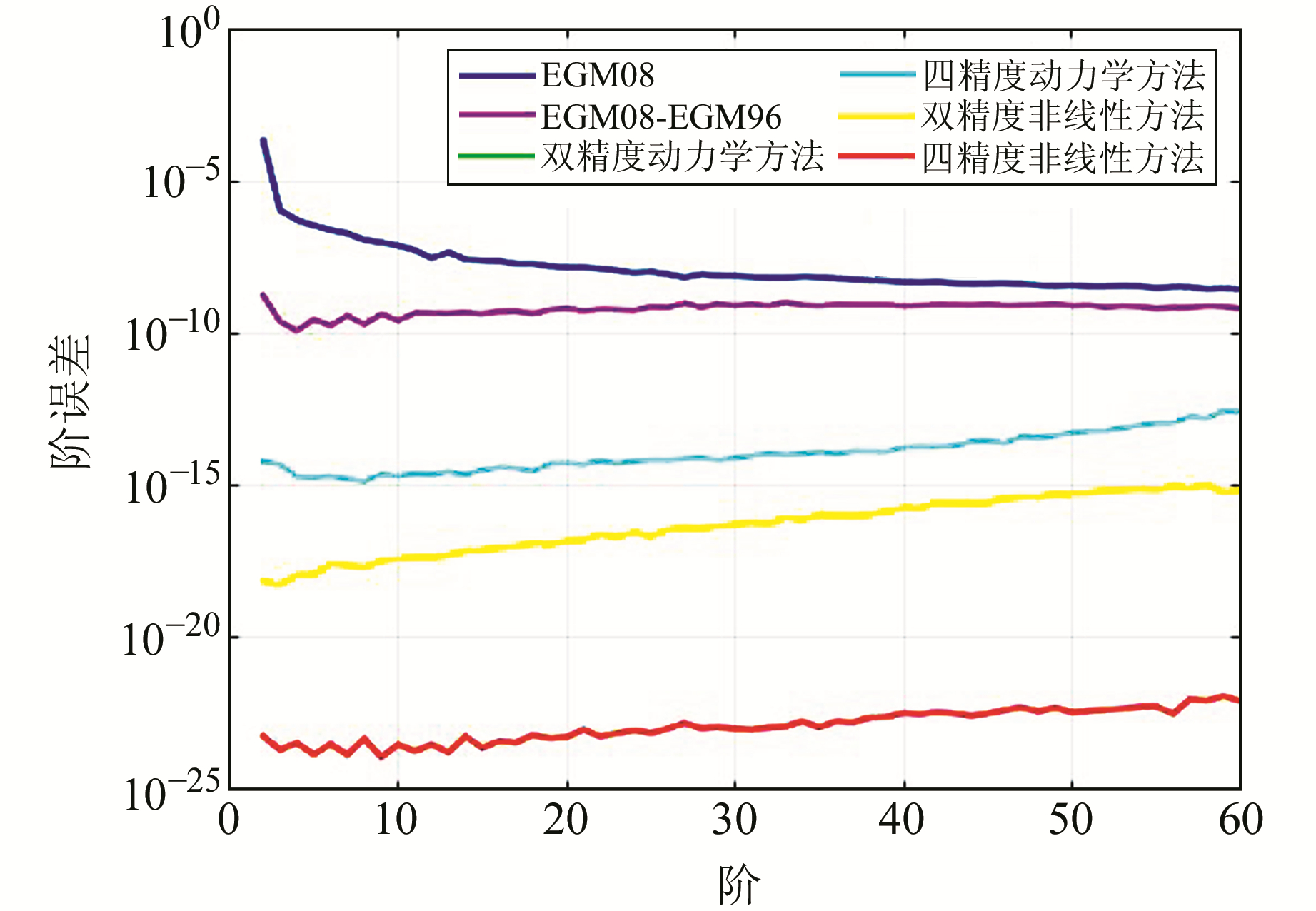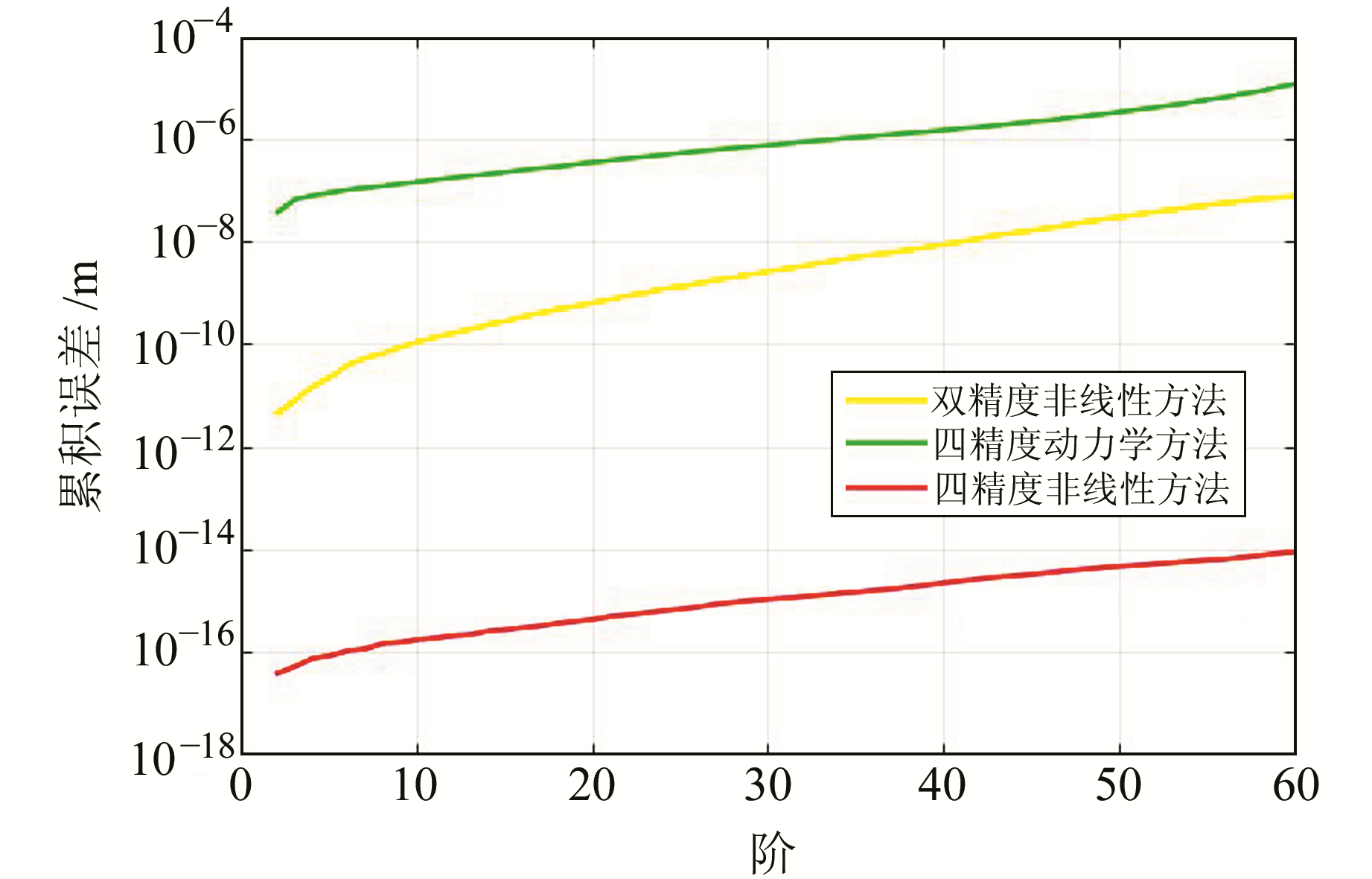﻿ 基于双精度与四精度的重力场解算精度分析文章快速检索 高级检索
 大地测量与地球动力学2020, Vol. 40Issue (1): 94-97, 110  DOI: 10.14075/j.jgg.2020.01.018### 引用本文ZHU Yongchao, WAN Xiaoyun, ZHOU Baoxing. Accuracy Analysis of the Double Precision and Quadruple Precision Gravity Field Solution[J]. Journal of Geodesy and Geodynamics, 2020, 40(1): 94-97, 110.### Foundation support

Doctoral Scientific Research Foundation of Shandong Jiaotong University, No.50004934; Key Research and Development Program of Shandong Province, No.2019GGX101043; National Natural Science Foundation of China, No. 41674026; Fundamental Research Funds for the Central Universities, No.2652018027.

### Corresponding author

WAN Xiaoyun, PhD, senior engineer, majors in satellite gravimetry, E-mail: wanxy@cugb.edu.cn.

### 第一作者简介

ZHU Yongchao, PhD, engineer, majors in satellite gravimetry, E-mail: zyc_20081989@126.com.

### 文章历史

1. 山东交通学院交通土建工程学院，济南市海棠路5001号，250357;
2. 中国地质大学(北京)土地科学技术学院，北京市学院路83号，100083

1 原理

 $\begin{array}{l} U = \frac{{GM}}{r}{\sum\limits_{n = 0}^\infty {\left( {\frac{R}{r}} \right)} ^n}\sum\limits_{m = 0}^n {({{\overline C }_{nm}}\cos m\lambda + } \\ \;\;\;\;\;\;{\overline S _{nm}}\sin m\lambda ){\overline P _{nm}}\left( {\cos \theta } \right) \end{array}$ (1)

 $\left\{ {\begin{array}{*{20}{c}} \begin{array}{l} {\overline P _{n, m}} = {f_3}({f_4}{\text{cos}}\theta {\overline P _{n - 1, m}} - {f_5}{\overline P _{n - 2, m}}),\\ m < n - 1 \end{array}\\ \begin{array}{l} {\overline P _{n, n - 1}} = {f_2}{\text{cos}}\theta {\overline P _{n - 1, n - 1}}, \\ m = n - 1 \end{array}\\ \begin{array}{l} {\overline P _{n, n}} = {f_1}\sin \theta {\overline P _{n - 1, n - 1}}, \\ m = n \end{array} \end{array}} \right.$ (2)

 $\left\{ {\begin{array}{*{20}{c}} {{f_1} = \sqrt {\frac{{2n + 1}}{{2n}}} }\\ {{f_2} = \sqrt {2n + 1} }\\ {{f_3} = \sqrt {\frac{{2n + 1}}{{\left( {n - m} \right)\left( {n + m} \right)}}} }\\ {{f_4} = \sqrt {2n - 1} }\\ {{f_5} = \sqrt {\frac{{\left( {n - m - 1} \right)\left( {n + m - 1} \right)}}{{2n - 3}}} } \end{array}} \right.$ (3)

${\overline C _{nm}}$${\overline S _{nm}}$为完全正规化后的nm次引力位系数，其值通过地面重力或者卫星精密轨道进行反演计算。利用卫星精密轨道解算重力场球谐系数的计算方法主要有动力学积分法、短弧长积分法、能量法等，本文选用动力学积分法。动力学积分法的公式推导思路是基于牛顿运动方程将卫星轨道方程线性化，以此建立卫星精密轨道与球谐位系数的关系式，其微分方程可用式(4)表达，由此推导出动力学积分方法的基本公式(5)

 $\left\{ {\begin{array}{*{20}{c}} {\frac{{{\rm{d}}\mathit{\boldsymbol{X}}}}{{{\rm{d}}t}} = F(t, \mathit{\boldsymbol{X}})}\\ {\mathit{\boldsymbol{X}}({t_0}) = {\mathit{\boldsymbol{X}}_0}} \end{array}} \right.$ (4)
 $\mathit{\boldsymbol{X}}(t) = {\mathit{\boldsymbol{X}}_{{\rm{ref}}}}(t) + \mathit{\boldsymbol{S}}(t)\delta \mathit{\boldsymbol{p}} + \mathit{\boldsymbol{Q}}(t)\delta \mathit{\boldsymbol{X}}({t_0})$ (5)

 $\begin{array}{l} \mathit{\boldsymbol{\dot \rho }}(t) = {{\mathit{\boldsymbol{\dot \rho }}}_0}(t) + {{\mathit{\boldsymbol{\dot Q}}}^{\rm{A}}}\left( t \right)\delta {\mathit{\boldsymbol{X}}^{\rm{A}}}\left( {{t_0}} \right) - \\ {{\mathit{\boldsymbol{\dot Q}}}^{\rm{B}}}\left( t \right)\delta {\mathit{\boldsymbol{X}}^{\rm{B}}}\left( {{t_0}} \right) + \left( {{{\mathit{\boldsymbol{\dot S}}}^{\rm{A}}}\left( t \right) - {{\mathit{\boldsymbol{\dot S}}}^{\rm{B}}}\left( t \right)} \right)\delta \mathit{\boldsymbol{p}} \end{array}$ (6)

2 算例 2.1 递推勒让德函数计算精度的比较

 $\begin{array}{l} {\varepsilon _n}\left( \theta \right) = \left| {2{{\left[ {{{\overline P }_n}\left( {{\text{cos}} \theta } \right)} \right]}^2} + } \right.\\ \left. {4{{\left[ {\sum\limits_{m = 1}^n {{{\overline P }_{n, m}}} ({\text{cos}})} \right]}^2} - \left( {2n + 1} \right)} \right| \end{array}$ (7)图 1 勒让德函数计算阶误差 Fig. 1 Degree errors of Legendre function computing表 1 双精度与四精度模式的用时比较 Tab. 1 The time comparison between double precision and quadruple precision
2.2 数值积分器在两种精度模式下的结果对比图 2 数值积分器计算误差 Fig. 2 Computational errors of numerical integrator
2.3 不同精度下解算重力场的对比图 3 不同精度下解算的重力场结果对比 Fig. 3 Comparison of the results of gravity field calculation with different accuracy图 4 不同精度下的累积大地水准面误差 Fig. 4 Accumulated geoid errors with different accuracy
3 结语

  许厚泽. 卫星重力研究:21世纪大地测量研究的新热点[J]. 测绘科学, 2001, 26(3): 1-3 (Xu Houze. Satellite Gravity Mission New Hotpoint in Geodesy[J]. Science of Surveying and Mapping, 2001, 26(3): 1-3 DOI:10.3771/j.issn.1009-2307.2001.03.001) (0)  Pavlis N K, Holmes S A, Kenyon S C, et al. The Development and Evaluation of the Earth Gravitational Model 2008 (EGM2008)[J]. J Geophys Res, 2012, 117: B04 406 (0)  吴星, 刘雁雨. 多种超高阶次缔合勒让德函数计算方法的比较[J]. 测绘科学技术学报, 2006, 23(3): 188-191 (Wu Xing, Liu Yanyu. Comparison of Computing Methods of the Ultra-High Degree and Order[J]. Journal of Geomatics Science and Technology, 2006, 23(3): 188-191 DOI:10.3969/j.issn.1673-6338.2006.03.010) (0)  王建强, 赵国强, 朱广彬. 常用超高阶次缔合勒让德函数计算方法对比分析[J]. 大地测量与地球动力学, 2009, 29(2): 126-130 (Wang Jianqiang, Zhao Guoqiang, Zhu Guangbin. Contrastive Analysis of Common Computing Methods of Ultra-High Degree and Order Fully Normalized Associated Legendre Function[J]. Journal of Geodesy and Geodynamics, 2009, 29(2): 126-130) (0)  于锦海, 曾艳艳, 朱永超, 等. 超高阶次Legendre函数的跨阶数迭代算法[J]. 地球物理学报, 2015, 58(3): 748-755 (Yu Jinhai, Zeng Yanyan, Zhu Yongchao, et al. A Recursion Arithmetic Formula for Legendre Functions of Ultra-High Degree and Order on Every other Degree[J]. Chinese Journal of Geophysics, 2015, 58(3): 748-755) (0)  于锦海, 万晓云. 计算Legendre函数导数的非奇异方法[J]. 测绘科学技术学报, 2010, 27(1): 1-3 (Yu Jinhai, Wan Xiaoyun. Non-Singular Formulate for Computing Derivatives of Legendre Functions[J]. Journal of Geomatics Science and Technology, 2010, 27(1): 1-3 DOI:10.3969/j.issn.1673-6338.2010.01.001) (0)  万晓云. 引力场梯度张量的非奇异公式推导[J]. 武汉大学学报:信息科学版, 2011, 36(12): 1 486-1 489 (Wan Xiaoyun. New Derivation of Nonsingular Expression for Gravitational Gradients Calculation[J]. Geomatics and Information Science of Wuhan University, 2011, 36(12): 1 486-1 489) (0)  Montenbruck O, Gill E. Satellite Orbits:Models, Methods and Applications[M]. Berlin: Springer-Verlag, 2000 (0)  Wenzel G. Ultra-high Degree Geopotential Models GPM98A, B and C to Degree 1 800[C].Joint Meeting of the International Gravity Commission and International Geoid Commission, Trieste, 1998 (0)  Wittwer T, Klees R, Seitz K. Ultra-High Degree Spherical Harmonic Analysis and Synthesis Using Extended Range Arithmetic[J]. J Geod, 2008, 82: 223-229 DOI:10.1007/s00190-007-0172-y (0)  Fukushima T. Numerical Computation of Spherical Harmonics of Arbitrary Degree and Order by Extending Exponent of Floating Point Numbers[J]. J Geod, 2012, 86: 271-285 DOI:10.1007/s00190-011-0519-2 (0)  Daras I, Pail R, Murböck M, et al. Gravity Field Processing with Enhanced Numerical Precision for LL-SST Missions[J]. Journal of Geodesy, 2015, 89(2): 99-110 DOI:10.1007/s00190-014-0764-2 (0)  Hekiskanen W A, Moritz H. Physical Geodesy[M]. San Francisco: W H Freeman, 1967 (0)  Tapley B D. Fundamentals of Orbit Determination[A]//Sanso F, Rummel R. Theory of Satellite Geodesy and Gravity Field Determination[M]. Heidelberg: Springer, 1989 (0)  于锦海, 朱永超, 孟祥超. CHAMP型卫星定轨顾及非线性改正的轨道扰动方程[J]. 地球物理学报, 2017, 60(2): 514-526 (Yu Jinhai, Zhu Yongchao, Meng Xiangchao. The Orbital Perturbation Differential Equations with the Non-Linear Corrections for CHAMP-Like Satellite[J]. Chinese Journal of Geophysics, 2017, 60(2): 514-526) (0)
Accuracy Analysis of the Double Precision and Quadruple Precision Gravity Field Solution
ZHU Yongchao1WAN Xiaoyun2ZHOU Baoxing1
1. School of Civil Engineering, Shandong Jiaotong University, 5001 Haitang Road, Jinan 250357, China;
2. School of Land Science and Technology, China University of Geosciences (Beijing), 83 Xueyuan Road, Beijing 100083, China
Abstract: In this paper, the precision of gravity field model under double precision and quadruple precision mode is compared and analyzed based on dynamics method, including the calculation of associated Legendre function, numerical integrator and gravity field inversion results. In terms of Legendre function calculation, part of the angle can be calculated in the double accuracy mode to the 1 900 order and overflow problems occur beyond this order. However, in quadruple precision mode any angle satisfies the accuracy requirement and the result is 8 orders of magnitude higher relative to the double precision mode. The quadruple precision model of numerical integrator Adams predictive correction method is 4 orders of magnitude higher than the double precision model. In terms of gravity field inversion, the inversion results of dynamic method are consistent in double and quadruple precision mode, and the accumulated geoid error calculating to order 60 is statistically 1.29×10-5 m, mainly because the linearized error of the dynamics method dominates the calculation error. The nonlinear dynamics method is 7 orders of magnitude higher than the double precision model in the quadruple precision model, and its accumulated geoid errors are respectively 8.92×10-15 m and 8.16×10-8 m.
Key words: quadruple precision; Legendre function; numerical integrator; dynamic integration method